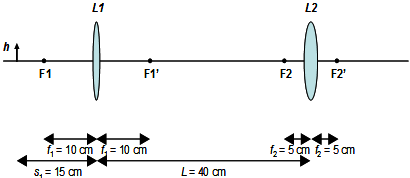## Draw the final image formed by two lenses, Physics

Assignment Help:

Draw the final image formed by two lenses:

An optical imaging system consists of two positive lenses (L1 and L2). L1 has a focal length f1 = 10 cm, and L2 has a focal length f2 = 5 cm. Two lenses are separated by L = 40 cm. An object (denoted by the vertical arrow) with height h is located in front of the first lens with a distance of 15 cm.(a) In the following diagram, draw the final image formed by these two lenses.

(b) Using the lens equation, please calculate the location of the final image with respect to the center of L2. Also what is the final magnification?

short article...

#### Half-life simulation, Aim : To obtain the half-life of two radioisotopes by...

Aim : To obtain the half-life of two radioisotopes by graphical means, using data from a simulated experiment. Theory :  Half-life( t ½ ) is the time it takes any particular ma

#### How and why cph theory does gainsay the relativity, how and why CPH Theory ...

how and why CPH Theory does gainsay the relativity? Answer; Never a CPH Theory gainsays the relativity. It can explain relativistic conceptions. Mainly; A. CPH Theory does c

#### Name the device used for data transmission, Name the device used for data t...

Name the device used for data transmission from single computer to an other Justify the name. Using this device draw the block diagram for data Communication and define it brief

#### Example on electrostatic shielding, Q. During lightning, it is safer to sit...

Q. During lightning, it is safer to sit inside car than in an open ground. Why? The metal body of the car offers electrostatic shielding where the electric field is zero. Thro

#### Electricity, What does it mean when a coulomb of charge has a certain numbe...

What does it mean when a coulomb of charge has a certain number of joules?

#### Analog electronics, current gam relashionship betweem alpha and betta

current gam relashionship betweem alpha and betta

#### Gauss divergence theorem, Using Gauss divergence theorem show that the volu...

Using Gauss divergence theorem show that the volume of a sphere of radius 'r' is 4⁄3 πr 3 .

the conical pendulum explzin in detail?

#### Theory vibration of magnetometer, explain?

explain?